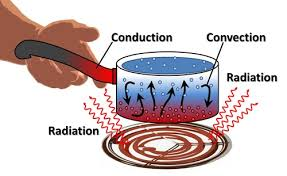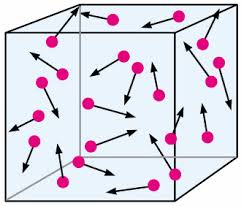Se muestran los artículos pertenecientes a Diciembre de 2017.

## 7th GRADE SCIENCE : Article 28 : Mechanical and Thermal energies, and temperature.* Activity 2.26 : Catapult involves kinetic, potential and mechanical energies.    ( 12/4/17   Monday )

2.26.a        In order to review what you learned last friday about the mechanical energy
involved when using a catapult, check this  PAPER  and answer the questions

( 15 points ).

2.26.b        Word search about mechanical energy and catapult.

( 5 points ).

2.26.c        Fill out the gaps on the text using the words given.

( 10 points ).

* Activity 2.27 : Showing Catapult. Kinetic, potential and mechanical energies.    ( 12/5/17   Tuesday )

2.27.a        Make the poster indicated by your teacher as in the example.
Follow his instructions and use the pictures given.

POSTER TO DO

Pics to cut for the poster

* Activity 2.28 :  Do the following activities about thermal energy.                    ( 12/6/17   Wednesday )
First you have a reviewing activity about catapults.

( 10 points ).

( 10 points ).

2.28.c        Friction force causing thermal energy
( 10 points ).

( Drawing )

( 10 points ).

* Activity 2.29 :  Radiation and convection. Thermal energy in a pot.           ( 12/7/17   Thursday )

PIC 2  about electromagnetic spectrum and energy.

( 10 points ).

2.29.b         Use the given material to show how water molecules move when
heating a pot on the heater.

Exemple to make.

( 20 points ).

2.29.         Fill in the suns shape crossword using the right words for each gap.

( 10 points ).

* Activity 2.30 :  Test about mechanical and thermal energies.                    ( 12/8/17   Friday )

2.30.a        Friday TEST about mechanical and thermal energies.

( 20 points ).

2.30.b        Heat and thermal energy exercise ( words-gaps to choose ).

( 10 points ).

2.30.c        Make these drawing in the given papers about thermal energy convection and
conduction.

Pics to draw.

( 10 points ).

## 7th GRADE SCIENCE : Article 29 : Particles in motion and kinetic energy.( 12/11/17   Monday )

* Activity 2.31 :  Particles motion, temperature and energy are highly related.

2.31.a        In order to understand the relationship between kinetic energy and particles
motion we do this Review that allows us to keep two concepts related.

( 10 points ).

2.31.b       Read the text and look carefully at the drawings describing the particles
movement.  After that, answer the suggested questions.

( 10 points ).

2.31.c       Complete the following tasks and the wordsearch.

( 10 points ).

( 12/12/17   Tuesday )

* Activity 2.32 :  Faster particles when hotter.

2.32.a        Today we finish the  tasks   we didnt yesterday.

( 10 points ).

( 12/13/17   Wednesday )

* Activity 2.33 :  Temperature, motion and kinetic energy.  Milk poster.

2.33.a        Use these pics from the papers given to prepare this poster :

( 20 points ).

( 10 points ).

( 12/14/17   Thursday )

* Activity 2.34 :  Energy and states, solid, liquid and gas. Temperature and kinetic energy.

2.34.a      Look up the following outlines and draw the proper particles in motion.

( 10 points ).

( 10 points ).

( 10 points ).

( 12/18/17   Monday )

* Activity 2.35 :  Particles and state matters structure.  Structure and energy.

2.35.a     Particles motion and structure related to energy changing.

a)  Look up this link and copy the structure particles take at
the different matter states (solid, liquid and gas) depending
on the given energy to the system.

b) Go to the following link and explain how energy
is involved into state matters changes. Write a summary about it.

( 10 points ).

2.35.b     Testing thermal energy.

Go to this link and do a short test about thermal energy.

( 10 points ).